﻿ 基于冗余计算约简的环扫SAR回波多GPU快速模拟
«上一篇文章快速检索 高级检索

 雷达学报2016, Vol. 5Issue (4): 434-443  DOI: 10.12000/JR150780

### 引用本文 [复制中英文]

[复制中文]
HU Chen, ZHANG Fan, LI Guojun, et al. Computation Reduction Oriented Circular Scanning SAR Raw Data Simulation on Multi-GPUs[J]. Journal of Radars, 2016, 5(4): 434-443. DOI: 10.12000/JR15078.
[复制英文]

### 文章历史

(北京化工大学信息科学与技术学院 北京 100029)
(北京遥感设备研究所 北京 100854)

Computation Reduction Oriented Circular Scanning SAR Raw Data Simulation on Multi-GPUs
(College of Information Science and Technology, Beijing University of Chemical Technology, Beijing 100029, China)
(Beijing Remote Sensing Equipment Research Institute, Beijing 100854, China)
Abstract: As a special working mode, the circular scanning Synthetic Aperture Radar (SAR) is widely used in the earth observation. With the increase of resolution and swath width, the simulation data has a massive increase, which boosts the new requirements of efficiency. Through analyzing the redundancy in the raw data simulation based on Graphics Processing Unit (GPU), a fast simulation method considering reduction of redundant computation is realized by the multi-GPUs and Message Passing Interface (MPI). The results show that the efficiency of 4-GPUs increases 2 times through the redundant reduction, and the hardware cost decreases by 50%, thus the overall speedup achieves 350 times than the traditional CPU simulation.
Key words: Circular scanning SAR     Raw data simulation     GPU parallel     Computing optimization
1 引言

2 环扫SAR回波模拟算法 2.1 成像几何模型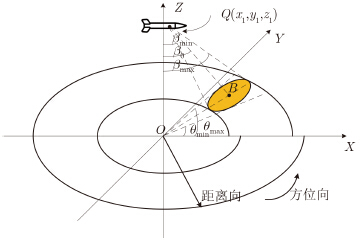图 1 环扫SAR成像几何 Fig. 1 Circular scanning SAR imaging geometry

 ${R_i} = \sqrt {{{({x_1} - {x_i})}^2} + {{({y_1} - {y_i})}^2} + {{({z_1} - {z_i})}^2}}$ (1)
 ${R'_i}=\sqrt {{{({x_1} - {x_i})}^2} + {{({y_1} - {y_i})}^2}}$ (2)

 ${{\beta }_{i}}=\arccos \left(\frac{{{z}_{1}}-{{z}_{i}}}{{{R}_{i}}}\right)$ (3)
 ${\theta _i} = \arccos \left( {\frac{{{{\rm{x}}_1} - {x_i}}}{R'_i}} \right)$ (4)

 ${\beta _{\min }} \le {\beta _i} \le {\beta _{\max }}$ (5)
 ${\theta _{\min }} \le {\theta _i} \le {\theta _{\max }}$ (6)
2.2 SAR回波模拟方法

SAR发射的chirp信号表达式如下：
 $s(\tau ) = {\rm{rect}}\left( {\frac{\tau }{{{T_{\rm{p}}}}}} \right)\exp \left( {{\rm{j2}}\pi {f_{\rm{c}}}\tau + {\rm{j}}\pi {k_{\rm{r}}}{\tau ^2}} \right)$ (7)

 $\begin{array}{*{20}{c}} {{s_{\rm{r}}}(t,\tau ) = \underbrace {\left\{ {{\rm{rect}}\left( {\frac{t}{{{T_{\rm{a}}}}}} \right) \cdot \exp \left( { - {\mathop{\rm j}\nolimits} \frac{{4\pi }}{\lambda }r(t)} \right)} \right\}}_{方位向}}\\ { \cdot \underbrace {\left\{ {{\rm{rect}}\left( {\frac{{\tau - \frac{{2r(t)}}{{\mathop{\rm c}\nolimits} }}}{{{T_{\rm{p}}}}}} \right) \cdot \exp \left[{{\mathop{\rm j}\nolimits} \pi {k_{\rm{r}}}{{\left( {\tau - \frac{{2r(t)}}{{\mathop{\rm c}\nolimits} }} \right)}^2}} \right]} \right\}}_{距离向}} \end{array}$ (8)

 $\begin{array}{*{20}{c}} {{s_{\rm{r}}}({t_n},\tau ) = \sum\limits_{n = 0}^T {{s_{\rm{a}}}({t_n},\tau )} \otimes s(\tau )}\\ { = \sum\limits_{n = 0}^T {{{\rm{F}}^{ - 1}}\left\{ {\left. {{\rm{F}}[{s_{\rm{a}}}({t_n},\tau )] \cdot S(\xi )} \right\}} \right.} } \end{array}$ (9)

 ${s_{\rm{a}}}({t_n},\tau ) = \sum\limits_i {\exp } \left\{ { - {\rm{j}}\frac{{4\pi }}{\lambda }{r_i}({t_n})} \right\} \cdot \left[{\delta \left( {\tau - \frac{{2{r_i}({t_n})}}{{\mathop{\rm c}\nolimits} }} \right)} \right]{\rm{ }}$ (10)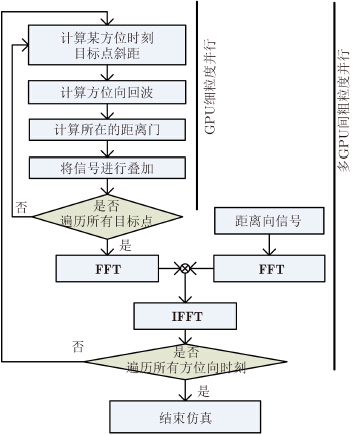图 2 SAR回波模拟仿真流程图 Fig. 2 Flowchart of SAR raw data simulation
3 基于MPI+多GPU冗余计算约简的优化方法 3.1 CUDA编程模型

CUDA (Compute Unified Device Architecture)是由显卡厂商英伟达推出的一种通用的并行计算架构，能够方便地将通用数值问题映射到GPU并行解决，因此GPU和传统算法的结合成为一个热点问题。

GPU与CPU在硬件架构上有着很大的不同，主要体现在逻辑控制、数据计算和存储体系方面。首先，由于芯片表面放置较少的逻辑控制单元，GPU的逻辑处理能力较差，因此在CPU+GPU异构系统中的逻辑控制部分通常由CPU完成。其次，GPU芯片表面比CPU芯片表面放置了更多的计算单元，因此GPU的计算能力优于后者。然后，GPU具有更加丰富的存储体系，每个线程都有自己的寄存器和本地存储器，多个线程构成一个线程块，每个线程块中的线程有共享的存储空间，多个线程块构成一个网格，网格中线程可以共享全局存储器、常量存储器和纹理存储器中的数据，GPU的存储模型如图 3所示。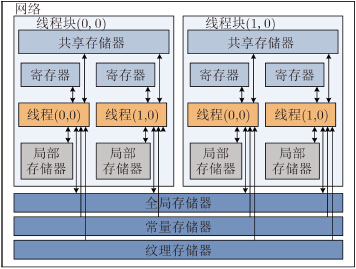图 3 GPU存储器模型 Fig. 3 GPU memory hierarchy

3.2 MPI并行编程库

CUDA用于完成单GPU卡的回波计算，对于多GPU卡的控制、任务调度，可以通过MPI完成。MPI全称消息传递接口，是一个并行的编程库，可以实现多机多核并行编程。在多GPU回波仿真中，任务的分发、收集都需要通过信息传递完成，而MPI是解决这一问题的最佳方法。MPI是基于消息传递机制的，可以灵活地进行仿真状态信息传递、仿真数据传输、多GPU计算进程控制等任务，便于进行不同粒度的并行，可以扩展到更多的GPU卡进行回波模拟工作，如图 4所示。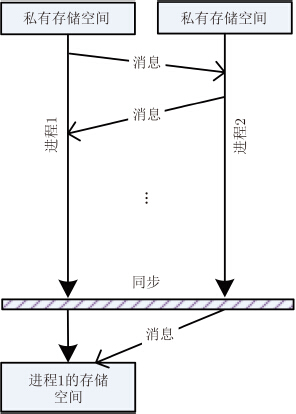图 4 MPI消息传递简图 Fig. 4 MPI message-passing diagram

3.3GPU并行回波模拟方法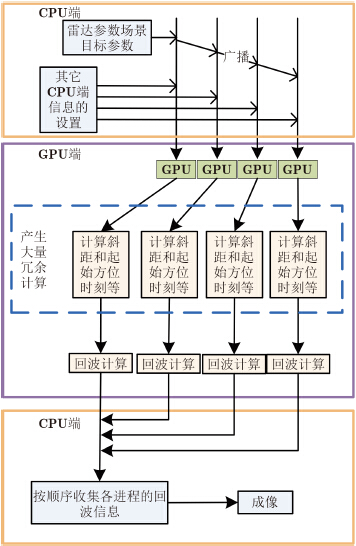图 5 多GPU的SAR回波模拟仿真流程 Fig. 5 Flowchart of multi-GPUs based SAR raw data simulation

(1) 读入雷达参数、场景目标参数等仿真基本信息；

(2) 将存入内存中的参数信息通过MPI主进程广播到其他MPI进程中；

(3) 通过不同的进程控制不同的GPU，并将各自内存空间中的参数信息和进程号传送到GPU显存中；

(4) 在GPU进行计算时，通过进程号、总进程数与总的方位向点数计算出该GPU卡在计算回波时的起始方位时刻和结束方位向时刻，也就是将整个方位向长度划分成了若干个小的方位向范围；

(5) 计算场景目标的斜距等成像几何信息，进而计算在该方位时刻的回波；

(6) 遍历该GPU所需遍历的所有方位向时刻，得到仿真回波数据子块；

(7) 计算完成后，将各自回波通过消息传递给主进程，CPU主进程进行回波的收集整理。

3.4 基于MPI的冗余计算约简

(1) 一个合成孔径范围相对于整个场景较小，但在进行波束脚印范围判断时却要计算场景中所有点的斜距、角度等成像几何信息，这样就产生了很大的冗余计算。

(2) 如果需要计算场景中所有点的成像几何信息，那么就需要将所有点的信息拷贝到GPU显存中，但实际上需要的只有该GPU计算任务中对应照射区域内的点信息，这样就产生了显存的浪费。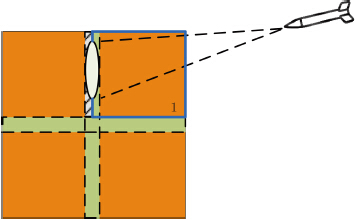图 6 雷达扫描分块边缘平面图 Fig. 6 Block edge diagram of radar scanning

 $\begin{array}{*{20}{r}} {S = \left( {\frac{{{\rm{height}}}}{{{\rm{MPI}}\_{\rm{NUM}}}}*2 + \frac{d}{2}} \right)}\\ {{\rm{*}}\left( {\frac{{{\rm{width}}}}{{{\rm{MPI}}\_{\rm{NUM}}}}*2 + \frac{d}{2}} \right)} \end{array}$ (11)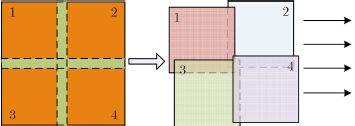图 7 MPI分块示意图 Fig. 7 MPI partitioning diagram

 ${\rm{Cpt\_total = (height}} * {\rm{width)}} * Cpt + echo\_Cpt$ (12)
 ${\rm{Cpt}}\_{\rm{total}}\_{\rm{pieces}} = \left( {\frac{{{\rm{height}}}}{{{\rm{MPI}}\_{\rm{NUM}}}}*2 + \frac{d}{2}} \right){\rm{*}}\left( {\frac{{{\rm{width}}}}{{{\rm{MPI}}\_{\rm{NUM}}}}*2 + \frac{d}{2}} \right){\rm{*Cpt}} + {\rm{echo}}\_{\rm{Cpt}}$ (13)
 $\begin{array}{*{20}{c}} {{N_{\rm{i}}} = \frac{{{\rm{Cpt}}\_{\rm{total}}}}{{{\rm{Cpt}}\_{\rm{total}}\_{\rm{pieces}}}} \approx \frac{{({\rm{height*width}}){\rm{*Cpt + echo}}\_{\rm{Cpt}}}}{{\frac{{{\rm{height*width}}}}{{{\rm{MPI}}\_{\rm{NU}}{{\rm{M}}^{\rm{2}}}}}{\rm{*4*Cpt + echo}}\_{\rm{Cpt}}}}}\\ { = {{\left( {\frac{{{\rm{MPI}}\_{\rm{NUM}}}}{{\rm{2}}}} \right)}^{2}} - \frac{{\left( {{{(\frac{{{\rm{MPI}}\_{\rm{NUM}}}}{{\rm{2}}}{\rm{)}}}^4} - {{(\frac{{{\rm{MPI}}\_{\rm{NUM}}}}{{\rm{2}}}{\rm{)}}}^2}} \right){\rm{*echo}}\_{\rm{Cpt}}}}{{({\rm{height*width}}){\rm{*Cpt}} + {{(\frac{{{\rm{MPI}}\_{\rm{NUM}}}}{{\rm{2}}})}^2}*{\rm{echo}}\_{\rm{Cpt}}}}} \end{array}$ (14)

4 实验结果分析 4.1 实验条件表 1 回波仿真参数 Tab. 1 Simulation parameters
4.2 加速效果分析表 2 不同场景下3种方法的计算时间对比 Tab. 2 Simulation time comparison of three algorithms in different scenes表 3 不同场景下多GPU和MPI分块的多GPU方法的加速比 Tab. 3 Speedup comparison of the multi-GPUs based method and the proposed method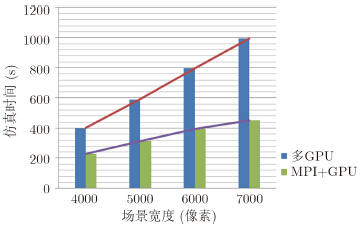图 8 多GPU和MPI+GPU的仿真总时间对比图 Fig. 8 Total simulation time comparison of the multi-GPUs based method and the proposed method表 4 不同场景下冗余计算约简的计算时间及加速比 Tab. 4 Performance analysis of the proposed method in time and speedup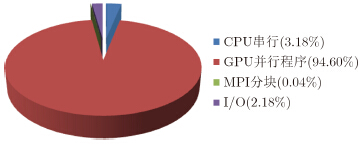图 9 7000×7000场景大小的运行时间比例图 Fig. 9 The running time scale map of the proposed method in 7000×7000 simulation area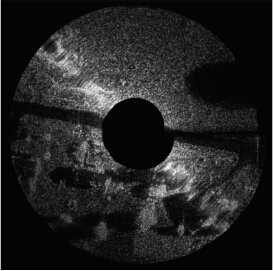图 10 本文方法仿真回波的成像结果 Fig. 10 The imaging result of the simulated raw data by the proposed method表 5 CPU方法和MPI分块的多GPU方法的仿真精度对比 Tab. 5 Accuracy analysis of the proposed methods
4.3 冗余存储优化分析表 6 相关硬件配置对比表 Tab. 6 Hardware configuration comparison
5 总结

  李天池, 周荫清, 蔡世学, 等. 机载环扫成像雷达数据处理方法研究[J]. 系统工程与电子技术, 2002, 24(7): 1-3. Li Tianchi, Zhou Yinqing, Cai Shixue, et al.. Study on the data processing methods for airborne circular scanning imaging radar[J]. Systems Engineering and Electronics, 2002, 24(7): 1-3.(1)  李静, 黄培康, 潘旭东, 等. 雷达下视环扫成像分辨率研究[J]. 系统工程与电子技术, 2009, 31(2): 315-318. Li Jing, Huang Peikang, Pan Xudong, et al.. Study on resolution for circular scanning imaging radar[J]. Systems Engineering and Electronics, 2009, 31(2): 315-318.(1)  李勇, 朱岱寅, 朱兆达. 环视 SAR 成像处理中的几何失真校正算法[J]. 南京航空航天大学学报, 2009, 41(2): 232-237. Li Yong, Zhu Daiyin, and Zhu Zhaoda. Geometric distortion correction algorithm for circular-scanning SAR imaging[J]. Journal of Nanjing University of Aeronautics & Astronautics, 2009, 41(2): 232-237.(1)  毛新华, 朱岱寅, 李勇, 等. 环视 SAR 几何失真校正误差分析及补偿技术研究[J]. 电子与信息学报, 2007, 30(11): 2706-2709.doi:10.3724/SP.J.1146.2007.00679. Mao Xinhua, Zhu Daiyin, Li Yong, et al.. Study on error analysis of geometric distortion correction and compensation techniques for circular-scan SAR[J]. Journal of Electronics & Information Technology, 2007, 30(11): 2706-2709. doi:10.3724/SP.J.1146.2007.00679.(1)  赵亮, 毛新华, 吴迪. 基于回波信号的环视 SAR 成像运动参数估计[J]. 数据采集与处理, 2014, 29(4): 590-596. Zhao Liang, Mao Xinhua, and Wu Di. Motion parameter estimate of circular-scanning SAR imaging based on echo signal[J]. Journal of Data Acquisition and Processing, 2014, 29(4): 590-596.(1)  孙兵, 周荫清, 李天池, 等. 环扫 SAR 的快速聚焦成像算法[J]. 北京航空航天大学学报, 2007, 33(7): 803-806. Sun Bing, Zhou Yinqing, LI Tianchi, et al.. Fast focused imaging algorithm for circular scanning SAR[J]. Journal of Beijing University of Aeronautics and Astronautics, 2007, 33(7): 803-806.(1)  苏宇, 齐向阳. 基于OpenMP的星载SAR回波信号并行仿真[J]. 中国科学院研究生院学报, 2008, 25(1): 129-135. Su Yu and Qi Xiangyang. OpenMP based space-borne SAR raw signal parallel simulation[J]. Journal of Graduate School of the Chinese Academy of Sciences, 2008, 25(1): 129-135.(1)  王曦爽, 黄立胜, 王贞松. 分布式星载SAR回波仿真的并行化计算研究[J]. 系统仿真学报, 2006, 18(8): 2097-2100. Wang Xishuang, Huang Lisheng, and Wang Zhensong. Research on parallel arithmetic of distribute spaceborne SAR ground target simulation[J]. Journal of System Simulation, 2006, 18(8): 2097-2100.(1)  张帆, 林殷, 洪文. 基于网格计算的SAR回波分布式仿真[J]. 系统仿真学报, 2008, 20(12): 3165-3167. Zhang Fan, Lin Yin, and Hong Wen. SAR echo distributed simulation based on grid computing[J]. Journal of System Simulation, 2008, 20(12): 3165-3167.(1)  Yu L, Xie X, and Xiao L. GPU-accelerated circular SAR echo data simulation of large scenes[C]. XXXIth URSI General Assembly and Scientific Symposium, Beijing, China, 2014: 1-4.(1)  Chapman W, Ranka S, Sahni S, et al.. Parallel processing techniques for the processing of synthetic aperture radar data on GPUs[C]. IEEE International Symposium on Signal Processing and Information Technology, 2011: 573-580.(1)  Zhu H, Xu H, and Feng L. Application of GPU for missile-borne SAR raw signal simulation[C]. International Conference on Artificial Intelligence, Management Science & Electronic Commerce, Zhengzhou, China, 2011: 2816-2820.(1)  Sheng H, Zhou M, Wang K, et al.. SAR echo simulation from numerous scattering cells based on GPU[C]. IET International Radar Conference, 2013: 1-5.(1)  秦洁, 张志敏. 基于图形处理单元架构的合成孔径雷达回波仿真实现与优化[J]. 科学技术与工程, 2014, 14(13): 85-89. Qin Jie and Zhang Zhimin. Implementation and optimization of SAR echo simulation based on GPU[J]. Science Technology and Engineering, 2014, 14(13): 85-89.(1)  Wang B, Zhang F, and Xiang M. SAR raw signal simulation based on GPU parallel computation[C]. IEEE Geoscience and Remote Sensing Symposium, Cape Town, South Africa, 2009: 617-620.(1)  Zhang F, Wang B, and Xiang M. Accelerating InSAR raw data simulation on GPU using CUDA[C]. IEEE Geoscience and Remote Sensing Symposium, Honolulu, Hawaii, USA, 2010: 2932-2935.(2)  Zhang F, Li Z, Wang B, et al.. Hybrid general-purpose computation on GPU (GPGPU) and computer graphics synthetic aperture radar simulation for complex scenes[J]. International Journal of Physical Sciences, 2012, 7(8): 1224-1234.(2)  Zhang F, Hu C, Li W, et al.. Accelerating time-domain SAR raw data simulation for large areas using multi-GPUs[J]. IEEE Journal of Selected Topics in Applied Earth Observations and Remote Sensing, 2014, 7(9): 3956-3966.(2)  黄立胜, 王贞松, 郑天垚. 基于FFT的快速SAR分布目标回波模拟算法[J]. 遥感学报, 2004, 8(2): 128-136. Huang Lisheng, Wang Zhensong, and Zheng Tianyao. A fast algorithm based on FFT used in simulation of SAR return wave signal[J]. Journal of Remote Sensing, 2004, 8(2): 128-136.(1)  Moving and Stationary Target Acquisition and Recognition (MSTAR) Public Dataset[OL]. https://www.sdms.afrl.af.mil/datasets/mstar/.(1)